# Printable Ten Frame Math SheetsJan 28, 2022By Printablee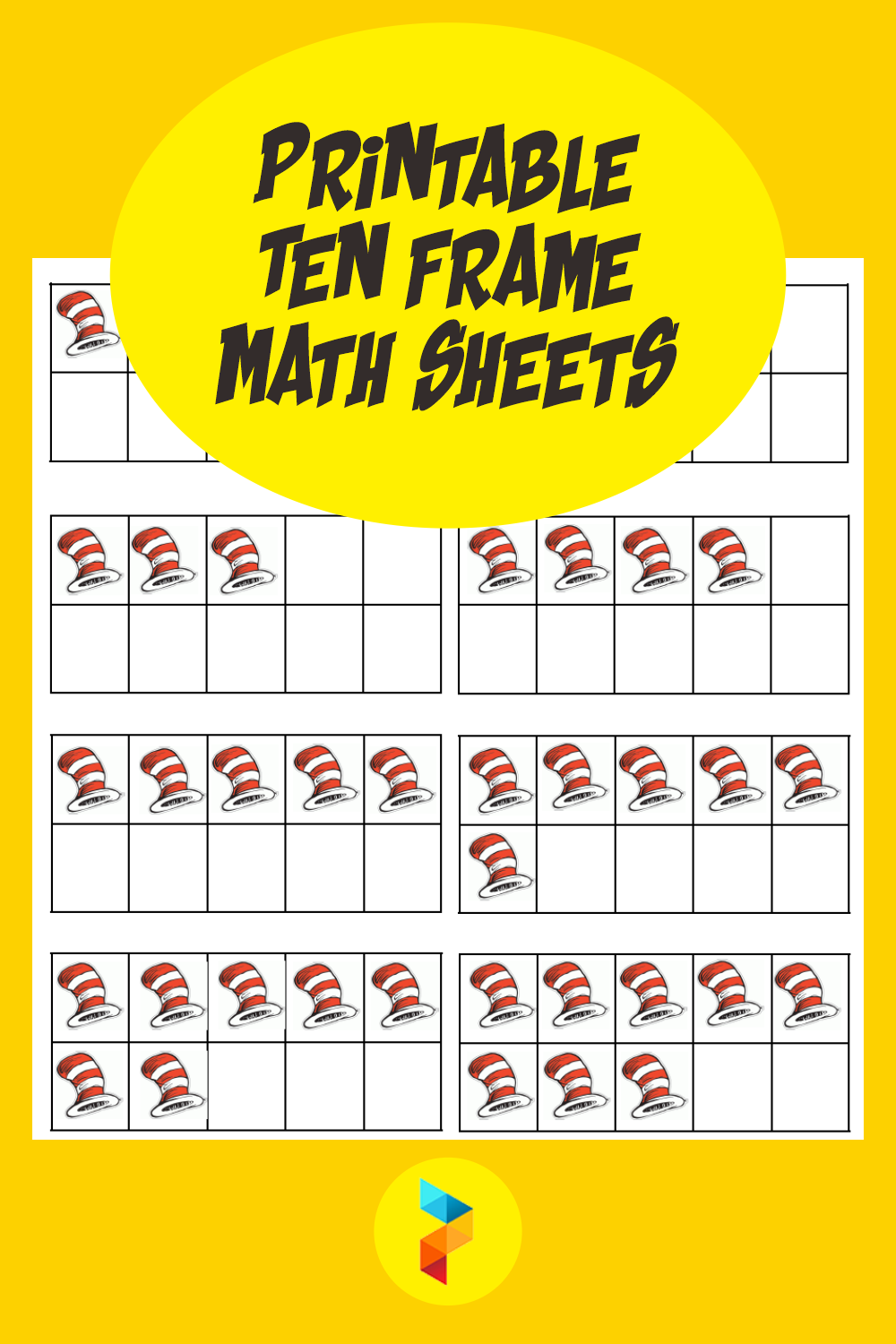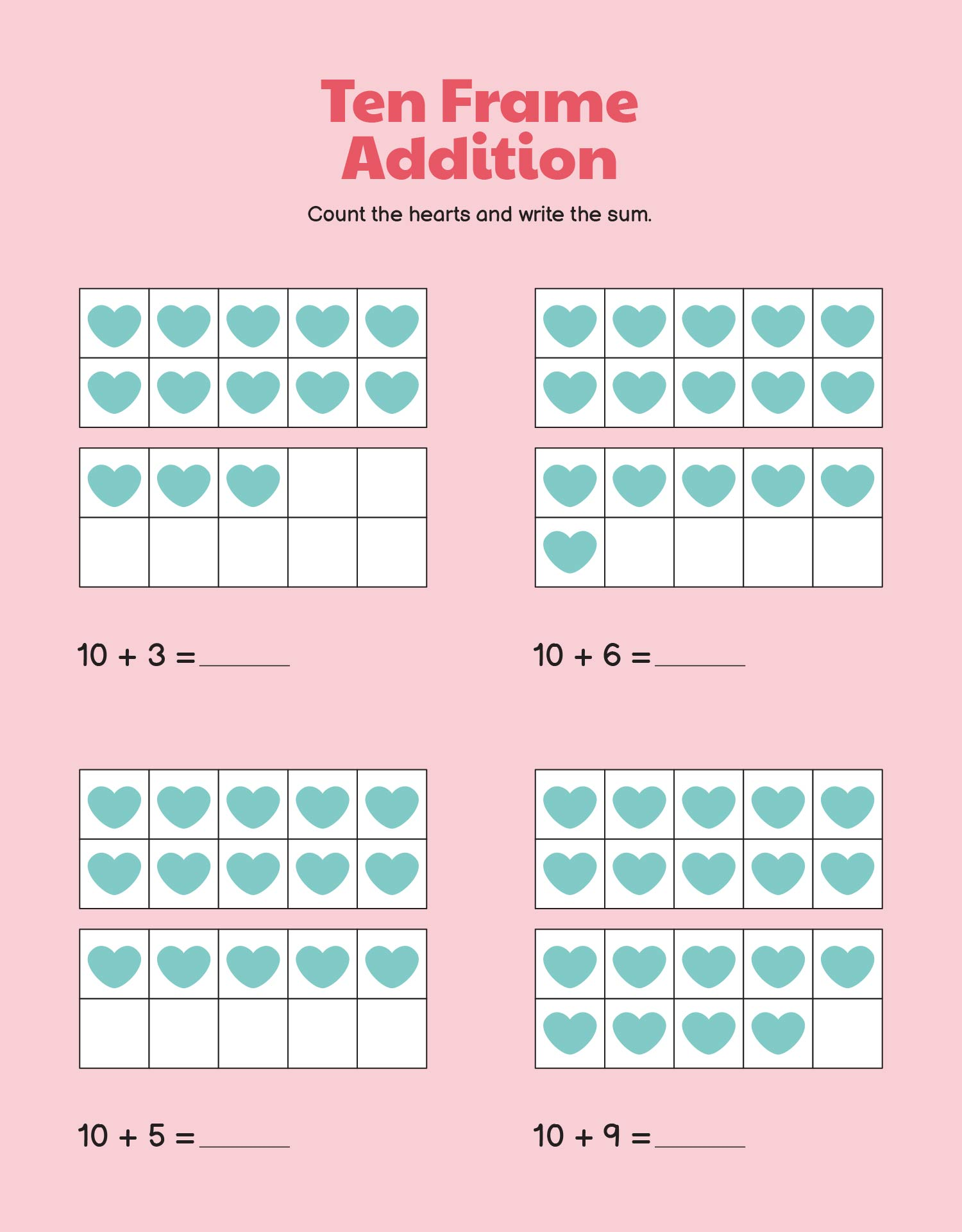### What is the purpose of a ten frame?

Ten frames become tools to understand mathematics. Ten frames are usually filled with a sequence of numbers or dots that function as numbers instead. The concept raised from ten frames is so that children can understand the value of a number. By understanding values, children will more easily solve basic math problems. Ten frames are usually used as a media for games to make it easier to understand.

### What is math computation?

In ten frames, basic mathematical problems can be answered with logic. Logic is found when the answer has a real reason because there is a settlement process that can be carried out coherently. An example is math computation which contains addition, subtraction, multiplication, division. Ten frames are involved in the process of finding answers. Ten frames as an explanation of the cause and effect of the placement of numbers in each frame. You can find the answer quickly if you practice diligently using ten frames. You can use ten frame math as sheets because it is easier to bring objects in sheets. You can tuck it in the pages of the textbook. With ten frame math sheets, you are more comfortable doing it when you have to move places like from school and then do it back at home.

### How can I improve my math computation skills?

People learn by doing. You can grow more with numbers if you continue to do activities that involve numbers. The more familiar you are, the more your memory and logic will be stimulated. Do it routinely Even when you still haven't found the answer, you still have to practice on your own and not run away with a calculator. Your teacher won't let you do math tests using a calculator, right? But drawing ten frames to complete your math test won't count as cheating.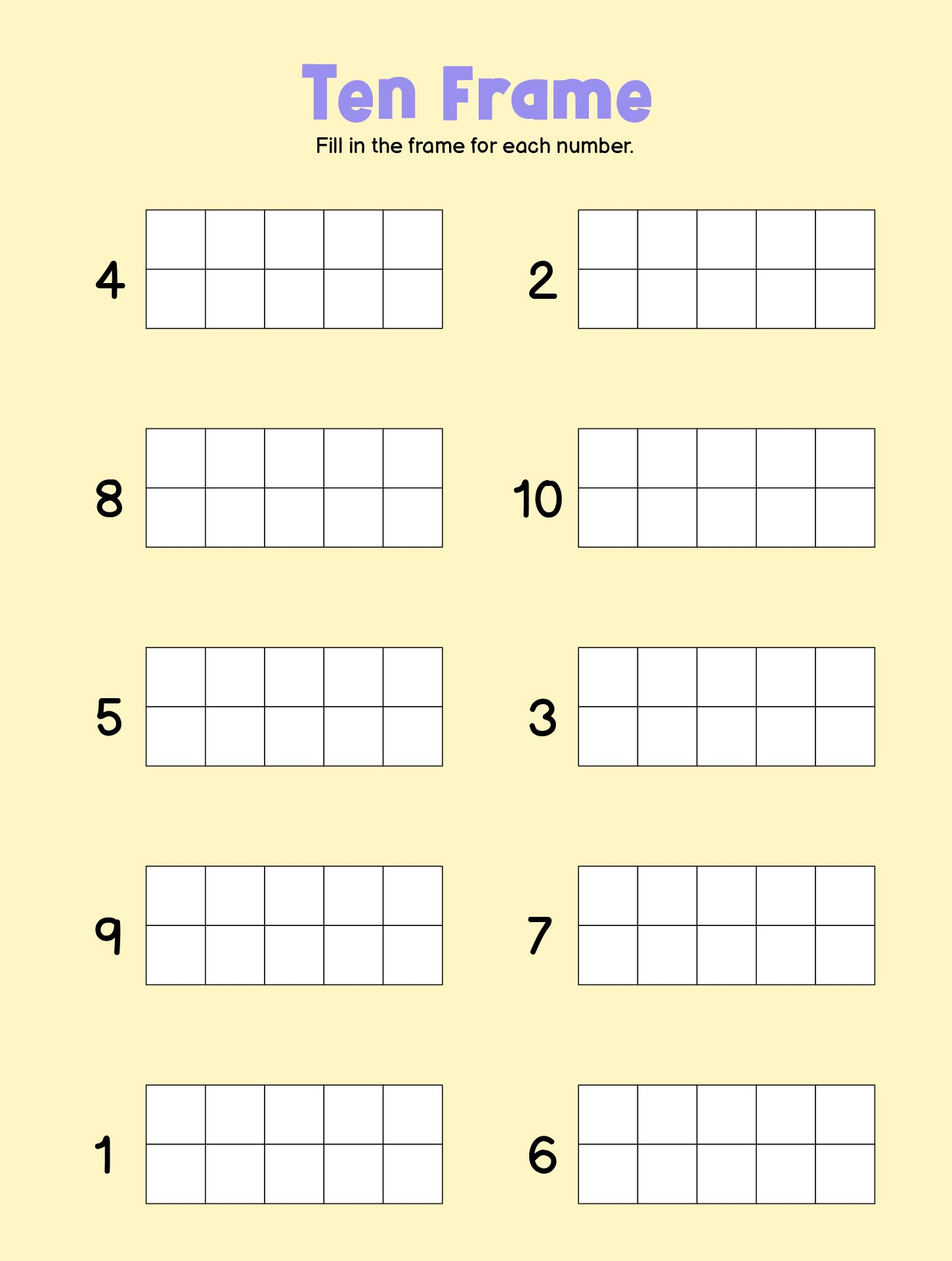We also have more printable frame you may like:
Blank Double Ten Frame Printable
Printable Ten Frame Activity
Ten Frame Template Printable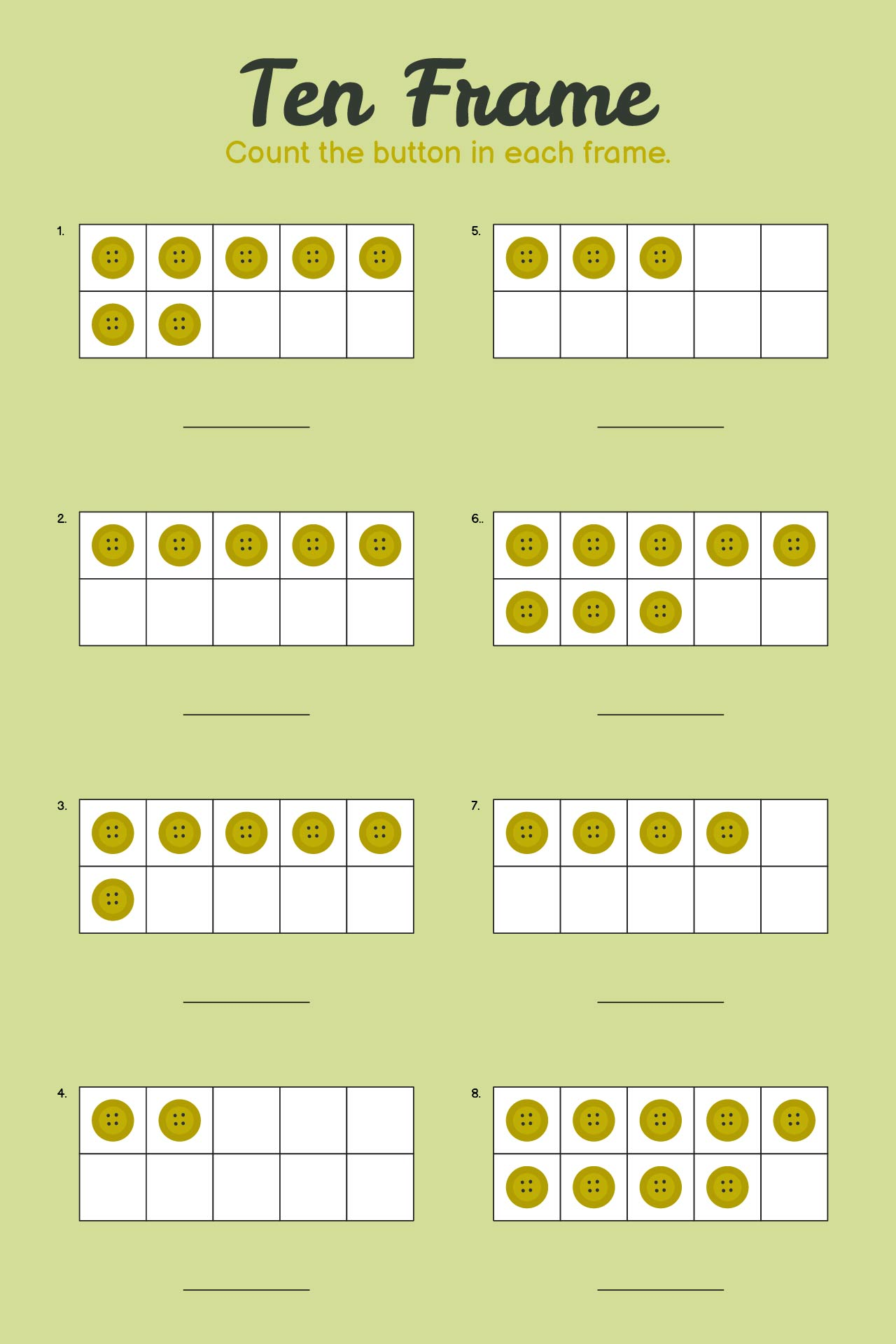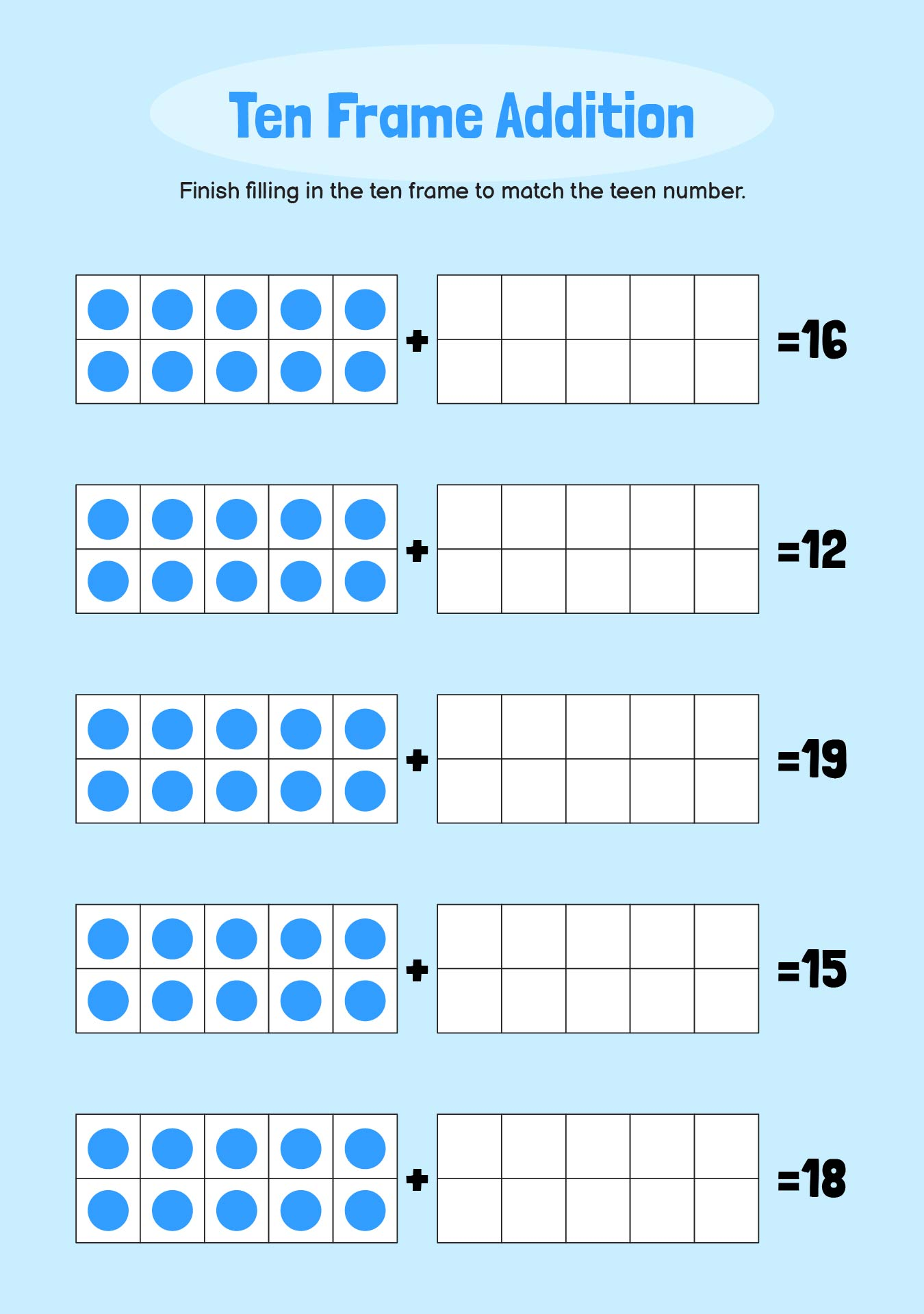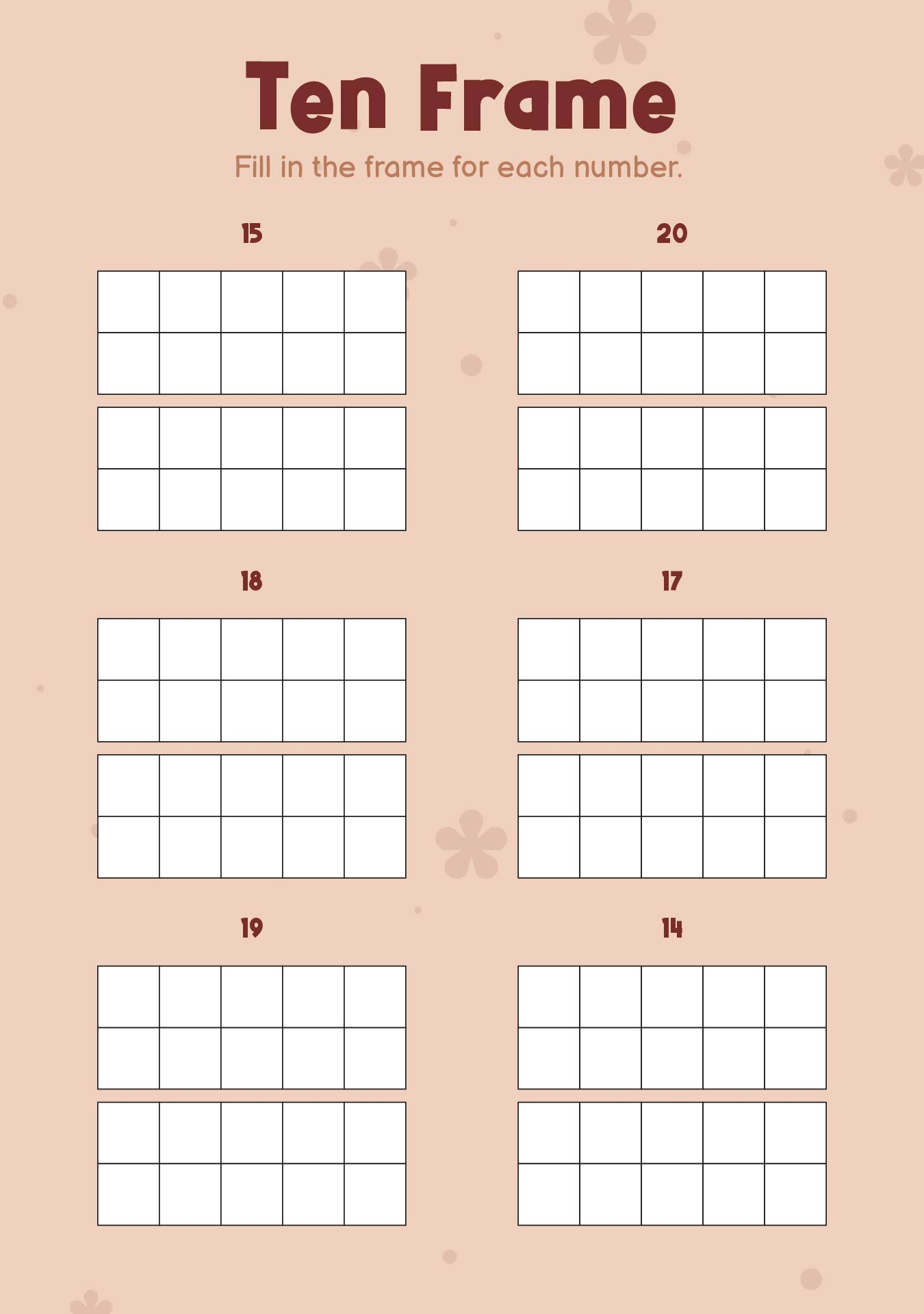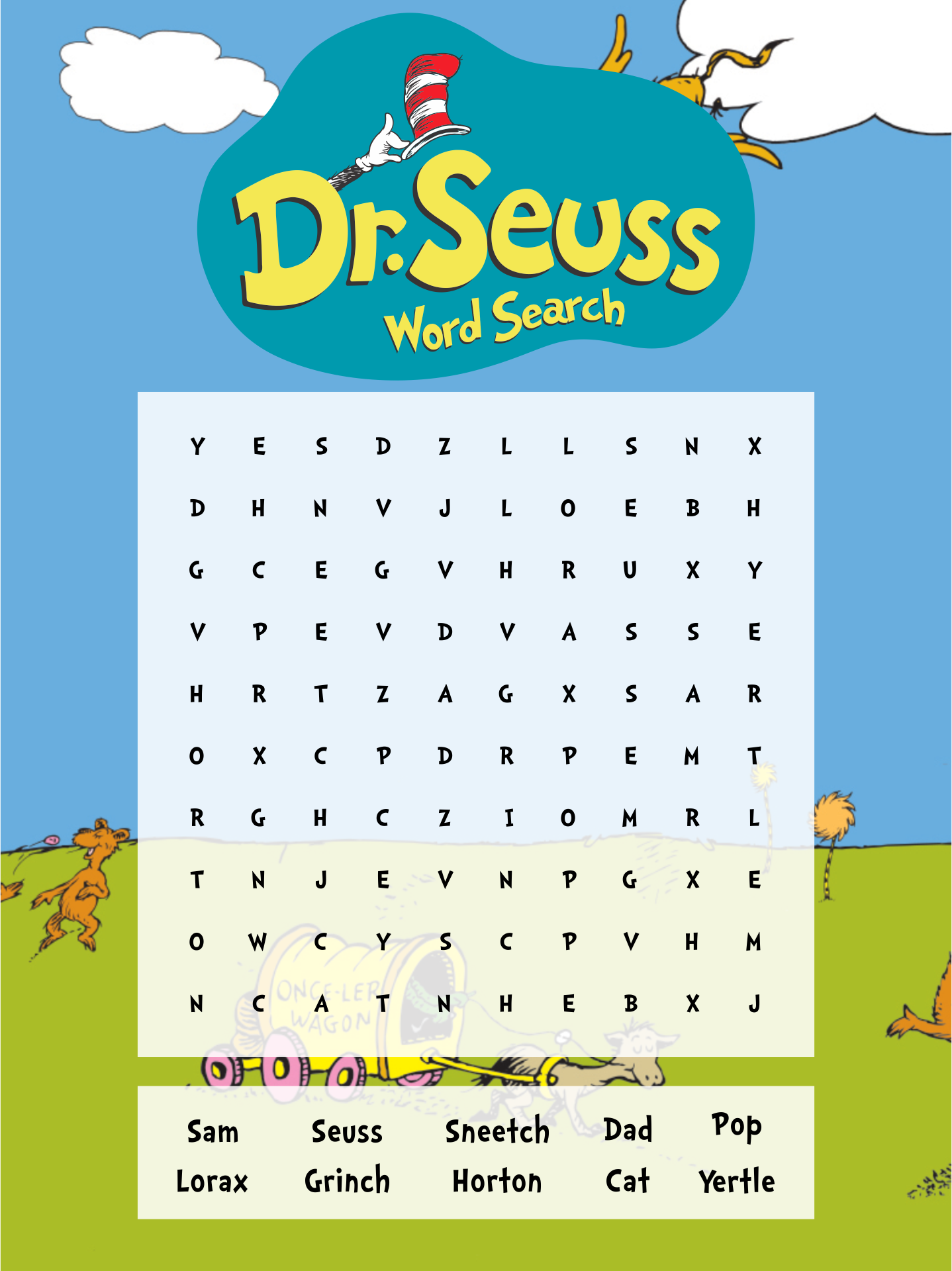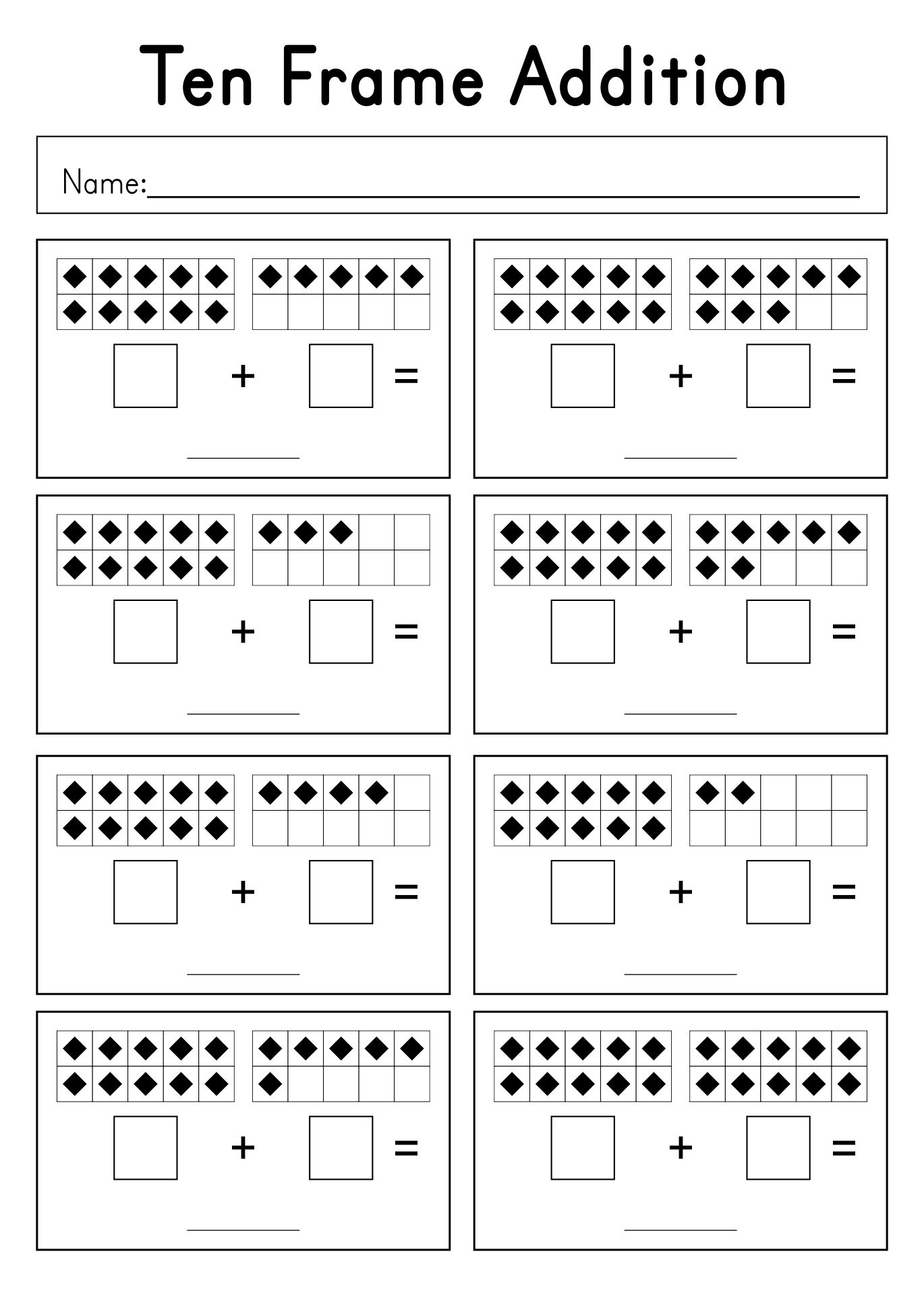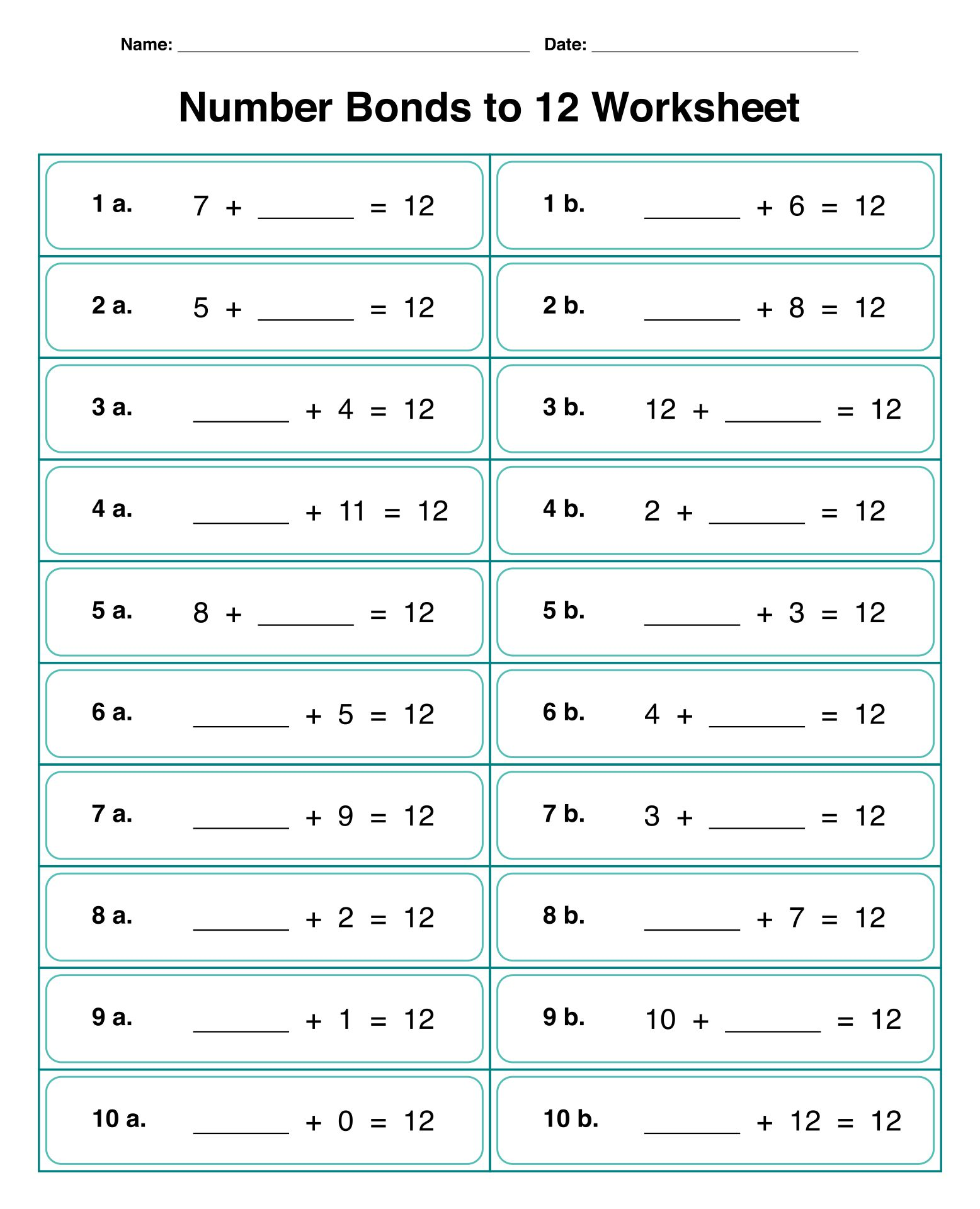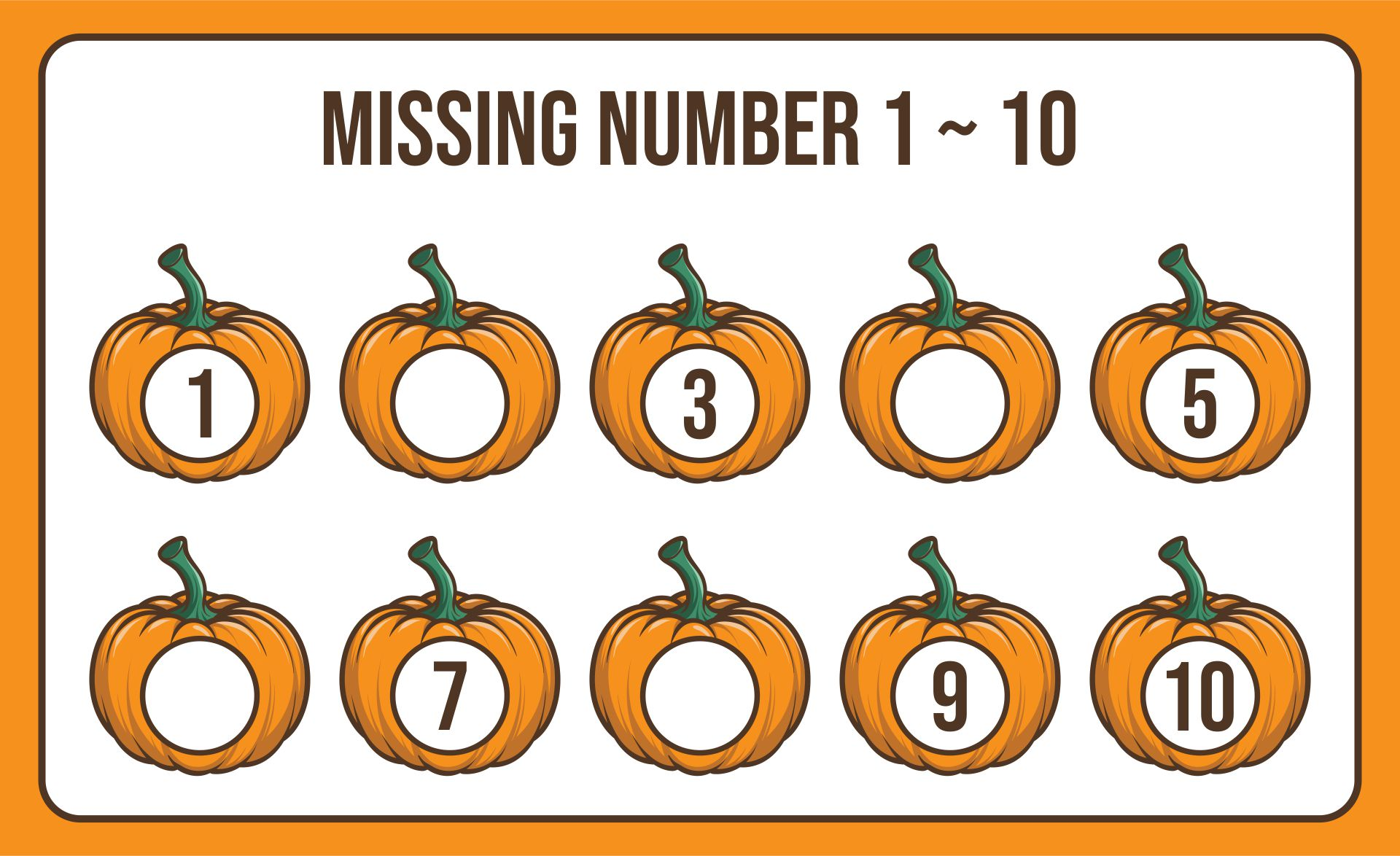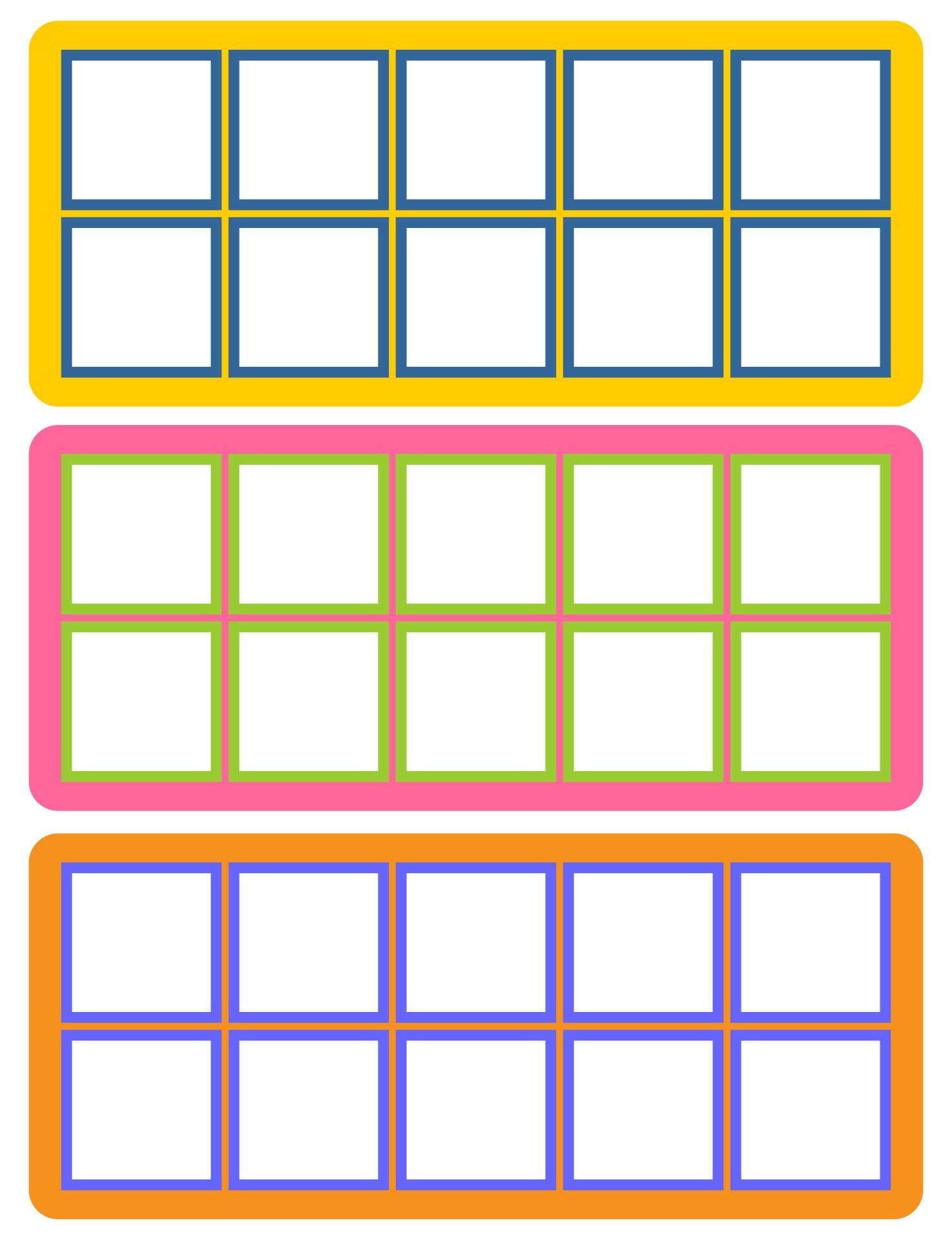### How do you show a subtraction in a ten frame?

Take a sheet with ten frames in it, we will learn to complete subtraction examples using ten frames. How to find the results of the number 5 reduced by number 2. First, fill in 5 frames. You can fill it with a dot, or just give a sign that the frame has contents. Remember, every frame has the same value. The key to subtraction is to prioritize larger numbers. After that, delete 2 frames. How many frames have contents? 3. Yes, it is the answer. Easy right? Now you can try it using other numbers. Problem solved.